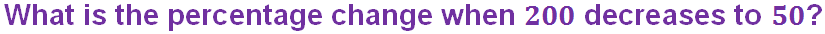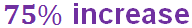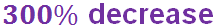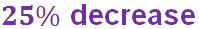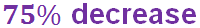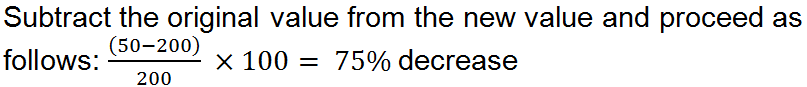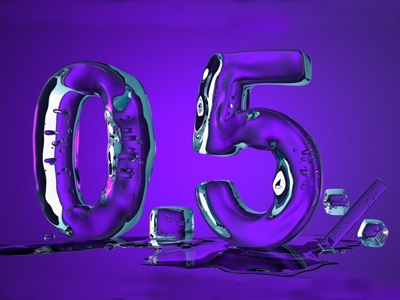Try to attain 100% in this quiz...

# Percentages (F)

A percentage is a special type of fraction, meaning ‘out of 100’, and even has a special symbol % to indicate it as a percentage. Percentage calculations are very similar to fractions, and don’t forget that ‘of’ means multiply! See how close to 100% you can get in this GCSE Maths quiz.

A common type of percentage calculation is to find the percentage of a quantity. This is useful if you see a sign saying ‘15% off’, as you can work out the saving you will make. The same technique can be used for increases – if you get a 5% wage rise, you can work out how much extra will be in your pay packet.

As percentages occur in everyday life, it is a good idea to be able to work simple percentages out in your head.

The basis for any mental calculation is 10%, as this is the fraction one-tenth, which is found by dividing by 10. You can then scale this result, so halve it for 5%, add 5% to 10% to find 15% etc.

In a sale, remember to subtract the discount amount from the original price to work out how much you still have to pay!

Another type of percentage calculation is percentage change, which occurs when something increases or decreases in value. Work out what the actual change in value is, then divide it by the ORIGINAL amount before multiplying the result by 100.

Question 1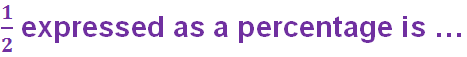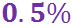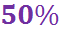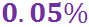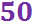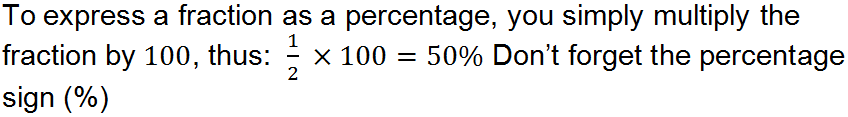Question 2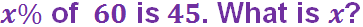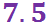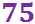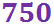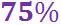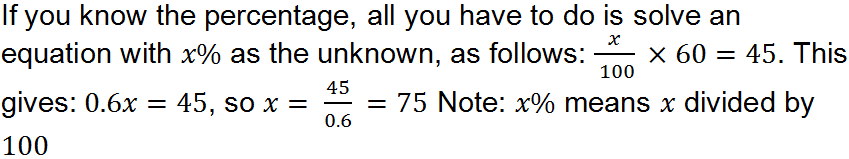Question 3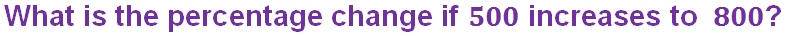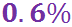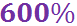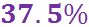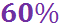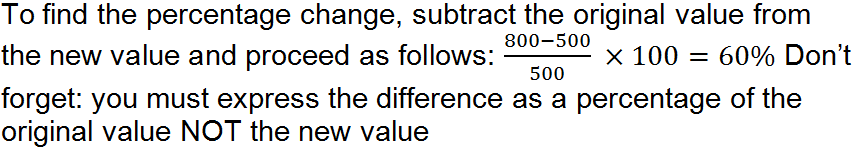Question 4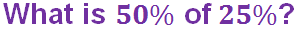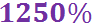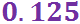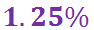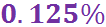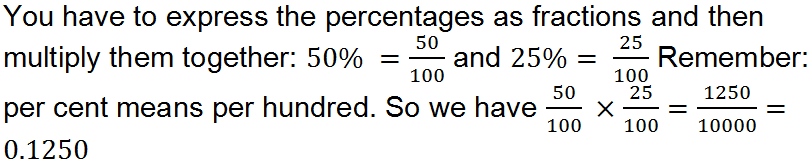Question 5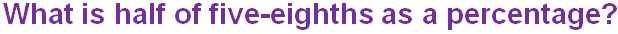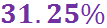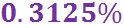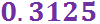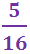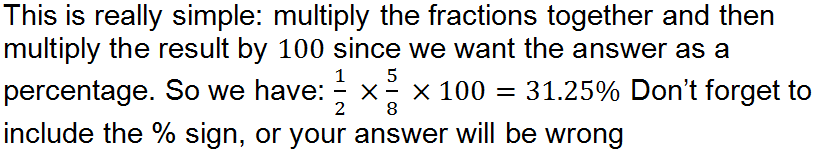Question 6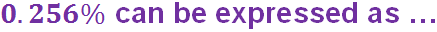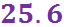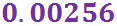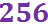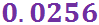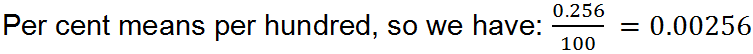Question 7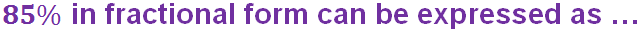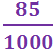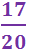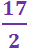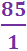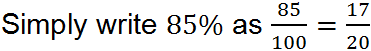Question 8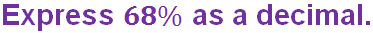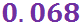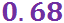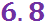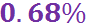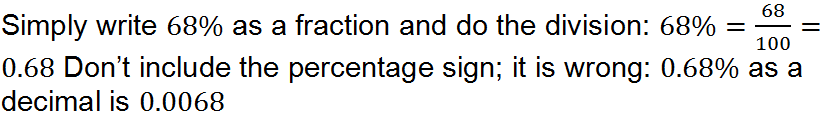Question 9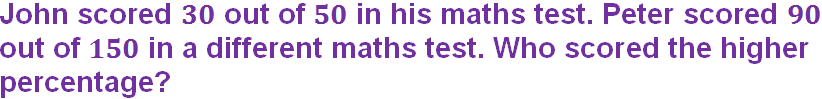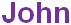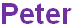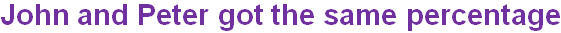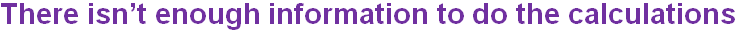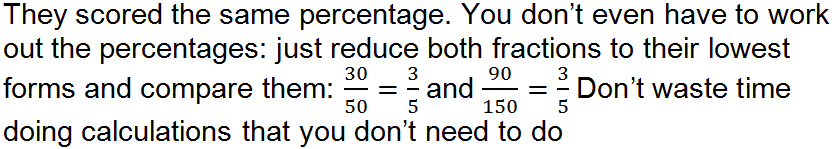Question 10